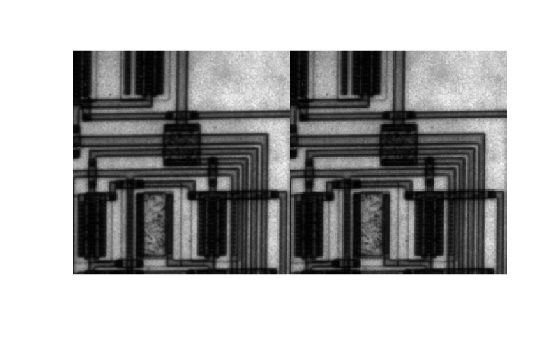# Resize an Image

This example shows how to resize an image using the `imresize` function.

Start by reading and displaying an image.

```I = imread("circuit.tif"); imshow(I)```### Specify Magnification Value

Resize the image, using the `imresize` function. In this example, you specify a magnification factor. To enlarge an image, specify a magnification factor greater than 1.

```magnificationFactor = 1.25; J = imresize(I,magnificationFactor);```

Display the original and enlarged image in a montage.

`imshowpair(I,J,method="montage")`### Specify Size of the Output Image

Resize the image again, this time specifying the desired size of the output image, rather than a magnification value. Pass `imresize` a vector that contains the number of rows and columns in the output image. If the specified size does not produce the same aspect ratio as the input image, the output image will be distorted. If you specify one of the elements in the vector as `NaN`, `imresize` calculates the value for that dimension to preserve the aspect ratio of the image. To perform the resizing required for multi-resolution processing, use `impyramid`.

```K = imresize(I,[100 150]); imshowpair(I,K,method="montage")```### Specify Interpolation Method

Resize the image again, this time specifying the interpolation method. When you enlarge an image, the output image contains more pixels than the original image. `imresize` uses interpolation to determine the values of these pixels, computing a weighted average of some set of pixels in the vicinity of the pixel location. `imresize` bases the weightings on the distance each pixel is from the point. By default, `imresize` uses bicubic interpolation, but you can specify other interpolation methods or interpolation kernels. You can also specify your own custom interpolation kernel. This example use nearest neighbor interpolation.

`L = imresize(I,magnificationFactor,"nearest");`

Display the resized image using bicubic interpolation, `J`, and and the resized image using nearest neighbor interpolation, `L`, in a montage.

`imshowpair(J,L,method="montage")`### Prevent Aliasing When Shrinking an Image

Resize the image again, this time shrinking the image. When you reduce the size of an image, you lose some of the original pixels because there are fewer pixels in the output image. This can introduce artifacts, such as aliasing. The aliasing that occurs as a result of size reduction normally appears as stair-step patterns (especially in high-contrast images), or as moire (ripple-effect) patterns in the output image. By default, `imresize` uses antialiasing to limit the impact of aliasing on the output image for all interpolation types except nearest neighbor. To turn off antialiasing, specify the `"Antialiasing"` name-value argument and set the value to `false`. Even with antialiasing turned on, resizing can introduce artifacts because information is always lost when you reduce the size of an image.

```magnificationFactor = 0.66; M = imresize(I,magnificationFactor); N = imresize(I,magnificationFactor,Antialiasing=false);```

Display the resized image with and without antialiasing in a montage.

`imshowpair(M,N,method="montage")`